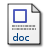# Efficient convex-elastic net algorithm to solve the Euclideantraveling salesman problem

Al-Mulhem, M. and Al-Maghrabi, T. (1998) Efficient convex-elastic net algorithm to solve the Euclideantraveling salesman problem. Systems, Man, and Cybernetics, Part B, IEEE Transactions on, 28.Preview
PDF
19KbMicrosoft Word
26Kb

## Abstract

This paper describes a hybrid algorithm that combines an adaptive-type neural network algorithm and a nondeterministic iterative algorithm to solve the Euclidean traveling salesman problem (E-TSP). It begins with a brief introduction to the TSP and the E-TSP. Then, it presents the proposed algorithm with its two major components: the convex-elastic net (CEN) algorithm and the nondeterministic iterative improvement (NII) algorithm. These two algorithms are combined into the efficient convex-elastic net (ECEN) algorithm. The CEN algorithm integrates the convex-hull property and elastic net algorithm to generate an initial tour for the E-TSP. The NII algorithm uses two rearrangement operators to improve the initial tour given by the CEN algorithm. The paper presents simulation results for two instances of E-TSP: randomly generated tours and tours for well-known problems in the literature. Experimental results are given to show that the proposed algorithm ran find the nearly optimal solution for the E-TSP that outperform many similar algorithms reported in the literature. The paper concludes with the advantages of the new algorithm and possible extensions

Item Type: Article August 1998 Publication Computer College Of Computer Sciences and Engineering > Information and Computer Science Dept Al-Mulhem, M. and Al-Maghrabi, T. 14378 KFUPM ePrints Admin 24 Jun 2008 16:33 12 Apr 2011 13:15

Repository Staff Only: item control page